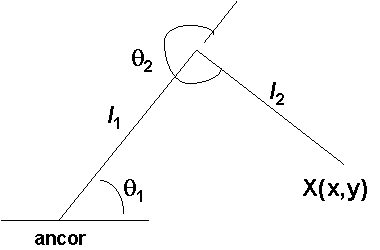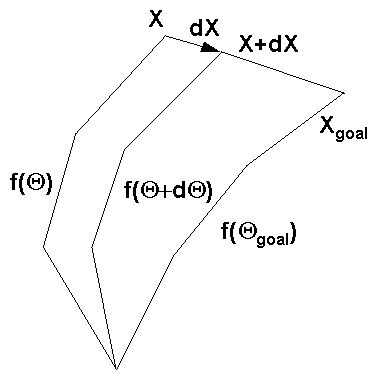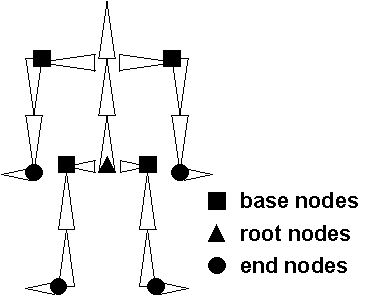# Animating Articulated Structures

(1995) Brian Lingard

## Introduction

Why would we want to animate articulated structures? Traditional animation (before computer graphics) depended heavily on the drawing of key frames to create character based animations and block out the desired motion. Early attempts at developing computer based animation systems also relied on key framing to specify motion. This technique requires a great deal of input from the animator. A better system would allow the animator to specify a motion sequence with only a few parameters. Thus newer animation systems use the techniques of forward kinematics and inverse kinematics to specify and control motion. The characters themselves are constructed out of skeletons which resemble the articulated structures found in robotics.

Thus the concept of synthetic actors has been introduced. Synthetic actors can be used to produce a more natural looking animation of human figures, which can even provide suggestions of feelings and emotions. The main benefit of these systems is that they usually do not require the use of key frames. Motion sequences can be specified with only a few parameters.

## Definitions

#### Kinematics

The study of motion independant of forces producing the motion.

#### Articulated figure

A structure consisting of rigid links connected at joints.

#### Degrees of freedom (DOF)

The number of independant joint variables specifying the state of the structure.

#### End Effector

The end of a chain of links, i.e. a hand or a foot.

#### State vector

The set of independant parameters which define a particular state of the articulated structure, thus the state vector Q is (Q1, Q2, ..., QN) where it has N degrees of freedom.

## Forward vs Inverse Kinematics

#### Forward kinematics

In forward kinematics the motion of all the joints in the structure are explicitly specified which yields the end effector position. The end effector position, X, is thus a function of the state vector of the structure, or:
```	X = f ( Q )
```
Thus the motion of the end effector is the accumlation of all the transformations that lead from the base of the structure to the end effector, as the hierarchy tree is descended, thus given Q derive X.

#### Inverse kinematics

In inverse kinematics (also known as "goal directed motion") the end effector's position is all that is defined. Given the end effector position, we must derive the state vector of the structure which produced that end effector position. Thus the state vector is given by:
```	     -1
	Q = f  ( X )
```

## Forward Kinematics

The figure below shows a hierarchy of two links where the links can only move in the plane of the web page. The end effector position is given as X(x,y) and the two joint angles are Q1 and Q2. Note that Q2 is relative to the orientation of link L1. Using geometric means (projecting each link onto the x and y axes) we can show that:
```  X = (l cos Q  + l cos (Q +Q ), l sin Q  + l sin (Q +Q ))
1     1    2      1  2    1     1    2      1  2
```
It must be noted that adding another joint will add at least another DOF. Though having more links will give more freedom of control, it may prove too complicated to achieve in practice.## Inverse Kinematics

Refering to our previous definition of inverse kinematics and the figure of the two links above, we wish to know what the joint angles Q1 and Q2 are given the end effector is at (x,y). Again using geometric means we show that:
```	Q = (Q1 , Q2)

-1    2    2     2     2
	Q  = cos   ((x  + y  - l   - l  ) / (2 l  l ))
2                      1     2         1  2

	Q  =  -(l  sin Q ) x + (l  + l  cos Q ) y
1       2      2        1    2      2
-----------------------------------
(l  sin Q ) y + (l  + l  cos Q ) x
2      2        1    2      2
```
Note that as DOF increases the problem on finding Q for a given X becomes underdefined. The state vector becomes a subspace of the possible solution space. Even for our two link example, there are two possible orientations of the links given a single end effector position.

Thus we must reduce the possible solution set by adding constraints on the system like energy conservation, momentum conservation, and movement constraints. Also using geometric means to find the inverse solution gets unbearably complicated as the DOF increases, thus we must find an alternate method.

## The Jacobian

Given X = f(Q) where X is of dimension n and Q is of dimension m, the Jacobian is the n x m matrix of partial derivatives relating differential changes of Q (dQ) to differential changes in X (dX), that is:
```	dX = J(Q) dQ
```
where the (i,j)th element of J is
```	      df
i
	J  = -----
ij   dx
j
```
but for use in computer graphics we usually divide everything by dt to give
```	.        .
	X = J(Q) Q
```
where dotX is velocity of the end effector which is itself a vector of six dimensions that include linear velocity and angular velocity, and where dotQ is time derivative of the state vector.

Thus the Jacobian maps velocities in state space to velocities in cartesian space. Thus at any time these quantities are related via the linear transformation J which itself changes through time as Q changes.

Why is this useful? Recall our inverse kinematics statement given as equation . If we localize around the current operating position and invert the Jacobian we get:

```	      -1
	dQ = J  (dX)
```
Thus we can iterate toward the goal over a series of incremental steps as shown in the figure below.If we recall our forward kinematics solution for the two link structure as shown previously (notation is abbreviated)

```	X = (l c  + l c   , l s  + l s  )
1 1    2 12    1 1    2 12
```
and differentiate using the chain rule
```	.         .          .    .        .          .    .
	X = (-l s Q  - l s  (Q  + Q ), l c Q  + l c  (O  + Q ))
1 1 1    2 12  1    2    1 1 1    2 12  1    2

.
| -l s  - l s       -l s   |  | Q  |
.   |   1 1    2 12       2 12 |  |  1 |
	X = |                          |  | .  |
| l c  + l c         l c   |  | Q  |
|  1 1    2 12        2 12 |  |  2 |
```
The 2x2 matrix on the left is the Jacobian and the column vector is the state vector Q. Note that this is only simple for very simple link hierarchies. Trying to differentiate for a structure of many links is tedious at best. Thus how do we go about constructing this Jacobian?

## Moving Axis Formula

To understand how to construct the Jacobian we must develop a system for referencing our chain of links. We can refer to the ith link's frame of reference as {i}, where we would have n links giving us a set of frames {{0}, {1}, ..., {n-1}, {n}}. Each frame {i} has its own coordinate system and we express the position and orientation of frame {i+1} relative to frame {i}, and frame {i} relative to frame {i-1} and so on. We must determine how to express the velocity of one frame relative to another frame given a specific frame of reference.If we consider two frames {i} and {j} whose origins are coincident and where {j} rotates with an angular velocity omega(ji) as shown in the figure above. The point Pj is fixed in {j} but appears to move with respect to {i}. To an observer in {i}, frame {j} appears to rotate by an amount omega(ji)dt over an incremental amount of time dt. [WATT, p.359] derives an expression for rotating a vector r about an axis n by an amount Q as:

```	Rr = r cos Q + (1-cos Q)n(n dot r) + (sin Q) n x r
```
where Rr is the rotated vector r. For incremental rotations we get
```	Rr = r + Q n x r
```
and we can substitute omega(ji)dt for Qn and Pj for r to get the incremental change of Pj as seen from {i} as
```	omega  dt x P
ji      j
```
and finally we divide by dt to get
```	V   = omega   x P
pi        ji    j
```
More generally, the origin of frame {j} could be moving with respect to frame {i} with linear velocity Vji and point P could be moving with velocity Vpj, these velocities are linear and may be simply added to get
```	V   = V   + omega   x P  + V
pi    ji        ji    j    pj
```
It is very important to note that the frame of reference used must be the same for all calculations, otherwise inconsistent values will result.

For the complete derivation of the following relations please refer to [WATT] pgs. 377 to 378. Given equation  we can now express the velocity of frame {k} relative to frame {i} via an intermediate frame {j} as
```	V   = V   + omega   x P   + V
ki    ji        ji    kj    kj
```
Using , we can show that the angular velocity of the end effector is
```		  n-1
	omega   = SUM omega
n0   i=1      i,i-1
```
or stated verbally is the sum of all the local angular velocities. A similar derivation for linear velocity yields
```	      n-1
	Vn0 = SUM omega     x P
i=1      i,i-1   ni
```
which says that the linear velocity of the end effector is the sum over all intermediate frames of the cross product of the local angular velocities with the vector from the end effector to the origina of that frame.

We can see how to use these relations, if we refer back to our two link example and apply equation . We see that the linear velocity of X is now V30 (for n=3) and is written out as

```	V   = omega   x P   + omega   x P
30        10    31        21    32
```
where we evaluate the following in frame {0}
```	P31 = (l1c1 + l2c12, l1s1 + l2s12)

P21 = (l2c12, l2s12)

.
omega   = ( 0, 0, 1)O
10              1
.
omega   = ( 0, 0, 1)O
21              2
```
Substituting and rearranging terms yields the same results as derived earlier by using differentiation.

The actual process of using these relations is fairly involved and beyond the scope of this article. The author hopes the reader has at least some appreciation for how the process of inverse kinematics works. Now let's look at a few example applications.

## Applications -- Human Figures

#### Skeletons

[WATT, pg 383] illustrates an example of constructing a skeleton using branching chains of links and which can have multiple roots. The figure shown below graphically depicts such a system.At each root or base node we can use inverse kinematics as an attachable engine. This engine governs the motion achieved in each subchain of links. A base node is in effect the end effector of a root node. Thus all combinations of limb flexion and spinal flexion can be achieved.

#### Life Forms

[CALV] describes a desktop system called Life Forms used to choreograph dance sequences and develop realistic animation of multiple figures. The system mixes keyframing, forward and inverse kinematic techniques.

The user (typically choreographers or animators) can block out a concept storyboard using the Stage editor. The user places characters on the stage, orients them, and specifies their motion using keyframes. This process is performed for all key scenes in the storyboard. Thus at this point only the character's movement around the stage is specified, not an individual character's movement of hands and legs.

For each figure being animated, the individual movements are contructed using a Sequence editor. The user can pick from menus or specify constraints on the limbs. Again key frames are used to speed up the entering of movements. Limbs can be moved via inverse kinematics just by grabbing a hand and moving it.

Finally multiple figures are combined using the Timeline editor to synchronize the individual movements with the movements of the other characters. Thus one character could be synchronized to duck just as another character is leaping over her head.

Life Forms supports various body models from stick figures to fully rendered characters. The system has been successfully used to help choreograph dance sequences for ballets and has been used by animators in creating elaborate dance sequences.

#### Legged Figures (walking)

[BRUD] presents the KLAW system (Keyframe-Less Animation of Walking) for creating walking sequences specified by only 3 parameters - forward velocity, step length, and step frequency. Only one of the 3 parameters needs to be specified. There are also 28 other attributes to individualize the walking.

[BRUD] notes in their article that walking can be broken down into two basic phases -- a swing phase and a stance phase. For walking there also exists a portion of the walking cycle where the stance phase of each leg overlaps. The motion of a leg is described separately for each of these two phases. It is relatively straight forward to derive the equations of motion when a leg consists of only two links. Complications arise when the foot and ankle joints are considered. The KLAW system also can take into account the pelvic list that most people exhibit when walking.

## Demo -- simple forward kinematics

The author of this article has developed a simple demo program for animating a single chain of links. The animation implements forward kinematics via a table of state vectors (joint angles) which are applied in a cycle of N frames. The joint angles are specified with respect to the world frame {0}. Future expansion of this demo is to include inverse kinematics, interactive grabbing and movement of the end effector. The demo software is available as a compressed tar file here (31.9K). This software makes use of the simple X library package called LIBSX. Please review the README file for information on obtaining LIBSX. The links included below are sample screen shots of the demo program in action.

Sample Screen Shot 1 (7.4K) - connected links

Sample Screen Shot 2 (6.9K) - unconnected links

Sample Screen Shot 3 (11.4K) - showing all joint positions

## References

[BRUD] Bruderlin and Calvert, "Goal-Directed, Dynamic Animation of Human Walking", Computer Graphics (Siggraph 1989), V23-3, pp. 233-242.

[CALV] Calvert et al, "Desktop Animation of Multiple Human Figures", IEEE Computer Graphics and Applications, May 1993, pp. 18-26.

[WATT] Watt and Watt, Advanced Animation and Rendering Techniques: Theory and Practice, Addison-Wesley, 1992, pp. 359, 369-394.

[MONH] Monheit and Bradler, "A Kinematic Model of the Human Spine and Torso", IEEE Computer Graphics and Applications, March 1991, pp. 29-38.

[SIMR] Simerman, "Anatomy of an Animation", Computer Graphics World, March 1995, pp. 36-43.

## Internet Sites

Some information on animation in general and other algorithms can be found on the following USENET groups:

lingard@wpi.wpi.edu